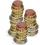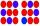# Division + multiplication principle - math problems

#### Number of problems found: 7

• Exchange € 100Find out how many ways you can exchange € 100 if you have an unlimited number of 50, 20, 10 and 5 euro banknotes. Use a method other than listing all options systematically.
• Twenty-fiveHow many are three-digit natural numbers divisible by 25?
• DivisionThe division has 18 members: 10 girls and six boys, two leaders. How many different patrols can be created if one patrol is two boys, three girls, and one leader?
• StacksAnnie has a total of \$ 702. The money must be divided into stacks so that each buyer has the same amount of dollars. How many options she has?
• CandyHow many ways can divide 10 identical candies to 5 children?
• No. of divisorsHow many different divisors have number 13 4 * 2 4?
• School tripThe class has 19 students. What different ways can students be accommodated in the hostel, where available 3× 2-bed, 3× 3-bed and 1× 4-bed rooms? (Each room has its unique number)

We apologize, but in this category are not a lot of examples.
Do you have an exciting math question or word problem that you can't solve? Ask a question or post a math problem, and we can try to solve it.

We will send a solution to your e-mail address. Solved examples are also published here. Please enter the e-mail correctly and check whether you don't have a full mailbox.

Division Problems. Multiplication principle - math problems.